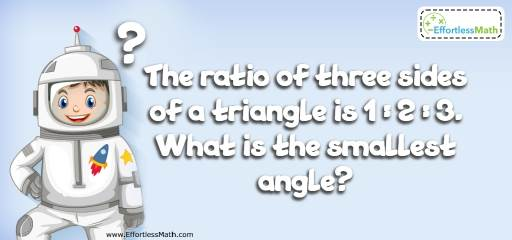# Geometry Puzzle – Challenge 62

This is a great mathematics puzzle to measure your basic math knowledge. Let's challenge your brain!## Challenge:

The ratio of three sides of a triangle is 1:2:3. What is the smallest angle?

### The Absolute Best Book to Challenge Your Smart Student!

The correct answer is 30 degree.

There is the same ratio for the angels. Therefore, the ratio of three angles of the triangle is also 1:2:3.
Let X be the smallest angle. Then, the next two angels are 2X and 3X.
X + 2X + 3X = 180 → 6X = 180 → X = 30
The smallest angel is 30 degree.

The Best Books to Ace Algebra

### What people say about "Geometry Puzzle – Challenge 62 - Effortless Math: We Help Students Learn to LOVE Mathematics"?

No one replied yet.

X
45% OFF

Limited time only!

Save Over 45%

SAVE $40 It was$89.99 now it is \$49.99